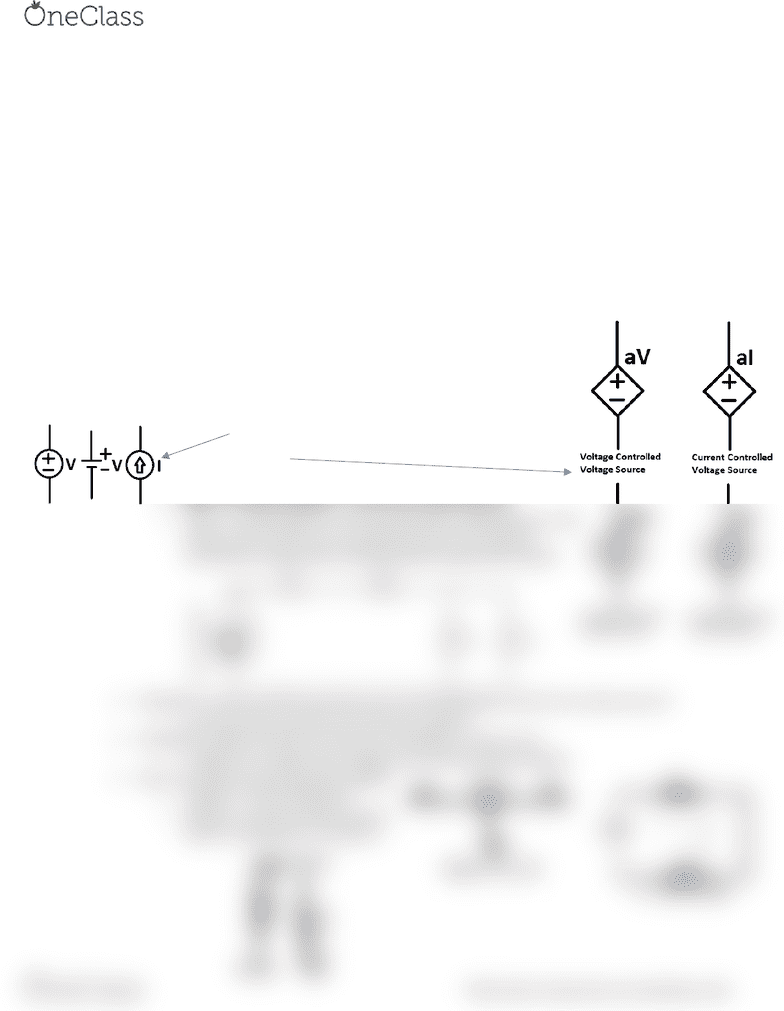Study Guides (380,000)
CA (150,000)
UW (6,000)
GENE (4)
Midterm

GENE123 Study Guide - Midterm Guide: Nodal Analysis, Direct Current, Linear Circuit

Department
General Engineering
Course Code
GENE123
Professor
Abdullah Mohammed
Study Guide
Midterm

This preview shows half of the first page. to view the full 2 pages of the document.GENE 123 Midterm Notes
Charge (Coulombs C) is an electrical property of the atomic particles
Current (Amperes A=C/S) is the rate of change of charge or charge per second
o Direct current is constant while alternating currents vary over time
Voltage (Volts V=J/C) is the energy required to move a unit of charge through an element
Energy (Joules J) is the capacity to do work
Power (Watts W=V*I=) is rate of absorbing or expending energy
Voltage drop occurs when you move from (+) to (-) and voltage rise occurs when you move from
(-) to (+)
Passive sign convention applies to passive elements and it states that the power is positive
(absorbed) when the current enters the positive polarity of the element, and negative (supplied)
when the current enters the negative polarity
Pico Nano Micro Mili Unit Kilo Mega Giga
P n µ m [-] k M G
10-12 10-9 10-6 10-3 1 103 106 109
Circuit elements
o Active elements supply power -> voltage/current sources
Independent does not care about other circuit elements
Dependent elements are affected by other circuit elements
o Passive elements absorb power -> resistor/capacitor/inductor
o Node is a connection where elements are joined together
o Series = no other path for current connected to node between circuit
elements (R1 and R2) versus parallel the ends of one element
connected to same node as ends of another element (R3 and R4)
Kirchhoff’s Curret La KCL says the su of currets floig i ad out of a ode sus to 0
o Current leaving is positive and entering is negative
Kirchhoff’s Voltage La KVL says the su of oltages i a loop is zero
o Voltage rise is negative and voltage drop is positive going clockwise
Oh’s La Passie sig coetio applies
o Voltage = Current*Resistance
o Resistor is a passive element whose
electric characteristic is resistance
find more resources at oneclass.com
find more resources at oneclass.com